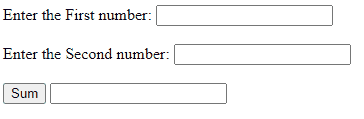Open in App
Not now

• Difficulty Level : Medium
• Last Updated : 19 Dec, 2022

Javascript comments explain the code design. They can also be used to prevent the execution of a section of code if necessary. Comments are ignored while the compiler executes the code. Comments are user-friendly as users can get explanations of code using comments.

Syntax:

`// For single line comment`
```/* For block of lines comment
...
...
*/```

Return Value: During the execution of code, comments are ignored.

Example 1: This example illustrates the single-line comment using //.

## Javascript

 ``

Example 2: This example illustrates the multi-line comment using /* … */

## Javascript

 ` ``    `

Example 3: Javascript comments can be used to prevent selective execution of code to locate problematic code or while testing new features. This example illustrates that commented code will never execute.

## HTML

 `<``body``>``    ``<``label``>Enter the first number``        ``<``input` `id``=``"num1"``>``    ````    ``<``br``><``br``>``    ``<``label``>Enter the first number``        ``<``input` `id``=``"num2"``>``    ````    ``<``br``><``br``>``    ``<``button` `onclick``=``"add()"``>``        ``Sum``    ````    ``<``input` `id``=``'sum'``>``    ``<``script``>``        ``function add() {``            ``var x, y, z;``            ``x = Number( document.getElementById("num1").value );``            ``y = Number( document.getElementById("num2").value );``            ``z= x +y;``            ``// Comment the code section``            ``//document.getElementById("sum").value = z;``        ``}``    `````

Output:Explanation: The sum is calculated but not displayed because the code to display sum is written in a comment and will not be displayed

My Personal Notes arrow_drop_up